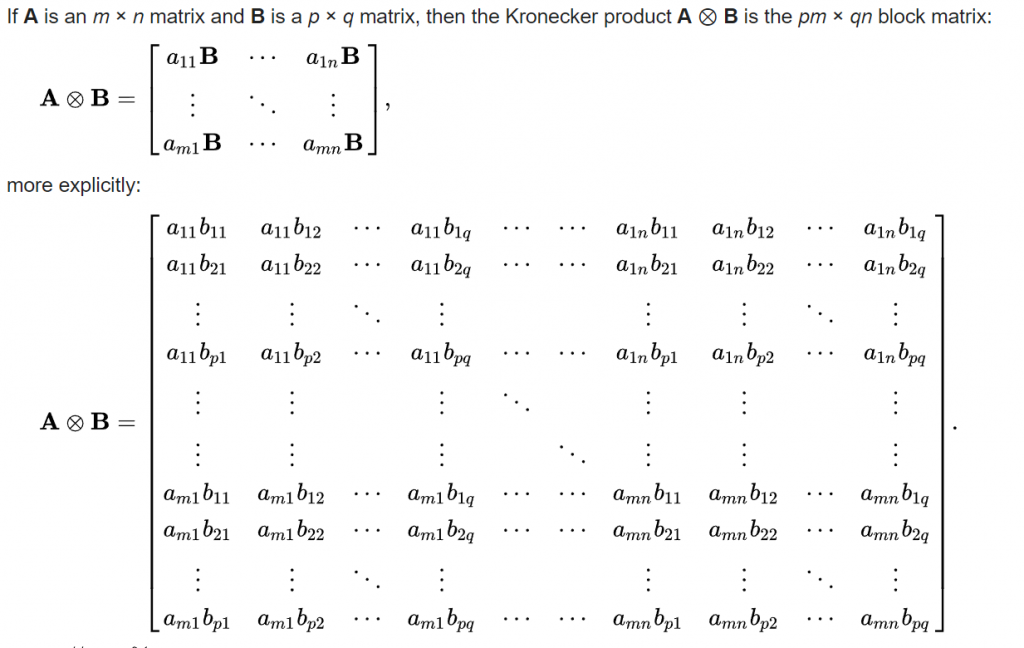# MATLAB repeating arrays (elementwise array replication, interleaved ‘repmat’)

Since MATLAB R2015b, there’s a new feature called repelem(V, dim1, dim2, ...) which repeats each element by dimX times over dimension X. If N (dim1) is scalar, each V is uniformly repeated by N times. If N is a vector, it has to be the same length as V and each element of N says how many times the corresponding element in V is repeated.

Here are some historical ways of doing it (as mentioned in MATLAB array manipulation tips)

The scalar case (repeat uniformly) can be emulated by a Kronecker product multiplying everything with 1 (self):

kron(V, ones(N,1))Just replace all the elements b with 1 so we are left with elements of A repeating the way we wanted

Kron method is conceptually smart but it has unnecessary arithmetic (multiply by 1). Nonetheless this method is reasonable fast until TMW finally developed a built-in function for it that outperforms all the tricks people have accumulated over decades.

The vector case (each element is repeated a different number of times according to vector N) is basically decoding Run-Length Encoding (RLE), aka counts to placements, which you can download maturely written programs on MATLAB File Exchange (FEX). There are a bunch of cumsum/diff/accumarray/reshape tricks but at the end of the day, they are RLE decoding in vectorized forms.

There’s a name for almost each recurring problem that we can think of in MALTAB. Before jumping in and implementing your for loop, ask around and try to find the right keyword/terms to describe your problem! >99.9% of the time your problem is not new!

The most odd-ball MATLAB algorithm scenario I’ve ever came across that requires original thought is the ‘Jenga Matrix‘ (I coined the name) while I was working at Stanford University Medical School as a research assistant for MADIT-CRT.

MATLAB’s OOP was not mature at that time, so dataset() objects didn’t surface. The reason for the ‘Jenga Matrix’ was to create ‘sparse cells’ which uses a sparse matrix with non-zero indices mapping to a cell vector so I can make a table (that’s approximately the guts of heterogenous data structure).

As I remove elements of the ‘sparse cell matrix’, I didn’t want holes in it to accumulate so I’ll have to periodically compact the underlying cell vector and shift the indices to reflect the indices after compacting. Normally if you have to mess with these kind of ingenious indexing algorithms, you are working on some generic abstractions/tools rather than the business logic itself.

There’s no ultimate correct way to implement something in MATLAB, but there are tons of bad ways that is strictly worse under all circumstances! Being smart with these little toy (Cody) problems like array manipulation do not really show practical proficiency in MATLAB. Anybody can spend a day or two to solve a genuinely new algorithm puzzle or just ask around in the forums if you run into it once in a blue moon. Who cares if you can do it 5 times faster if it’s just <1% of the development time?

Most of your time should be spent on using MATLAB to succinctly and intuitively describe your business logic (which requires exploring and understanding your project requirements deeply), and hide the boring background work with generic abstractions (e.g. RDBMS and RLE)! People should be able to read your function and variable names and form a clear picture of what your codebase is trying to achieve instead of stumbling over smart-ass idioms that’s not immediately obvious (which should buried in the lowest level of generic tool functions if you had to develop it in-house).

Even a mathematician in Linear Algebra using MATLAB for 40 years doesn’t mean he’s good at MATLAB! The real MATLAB skills are keeping up with MATLAB has to offer for a variety of scenarios relevant to the task at hand (or know enough abstract concepts like functional programming, OOP, database, etc, to be able to find out the right tools quickly), which is a hell lot of knowledge considering MATLAB covered most common scenario imaginable (the vast majority of MATLAB users aren’t aware of the full offerings and used MATLAB the wrong/hard way)!

54 total views,  1 views today

Subscribe
Notify of